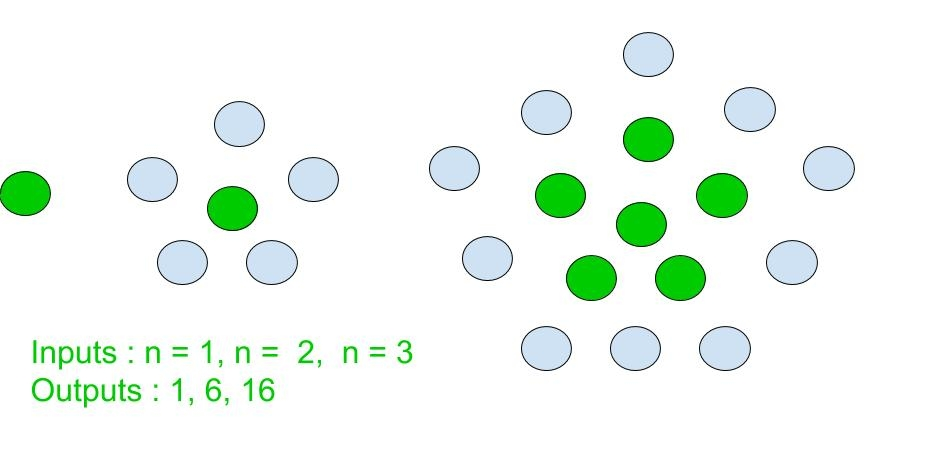GFG App
Open AppBrowser
Continue

Given an integer n, find the nth Centered pentagonal number.

A Centered Pentagonal Number is a centered figurate number that represents a pentagon with a dot in the center and other dots surrounding it in pentagonal layers successively [ Source: Wiki ]Few Centered pentagonal Number are :
1, 6, 16, 31, 51, 76, 106, 141, 181, 226, 276, 331, 391………………..

Examples :

```Input : 3
Output : 16

Input : 9
Output : 181```

Approach:
Centered pentagonal for n-th term is given by :

``

Basic implementation of the above approach :

## C++

 `// Program to find nth` `// Centered pentagonal number.` `#include ` `using` `namespace` `std;`   `// centered pentagonal number function`   `int` `centered_pentagonal_Num(``int` `n)` `{` `    ``// Formula to calculate nth` `    ``// Centered pentagonal number` `    ``// and return it into main function.`   `    ``return` `(5 * n * n - 5 * n + 2) / 2;` `}`   `// Driver Code` `int` `main()` `{` `    ``int` `n = 7;` `    ``cout << n << ``"th Centered pentagonal number: "``;` `    ``cout << centered_pentagonal_Num(n);` `    ``return` `0;` `}`

## C

 `// C Program to find nth` `// Centered pentagonal number.` `#include `   `// centered pentagonal number function` `int` `centered_pentagonal_Num(``int` `n)` `{` `    ``// Formula to calculate nth` `    ``// Centered pentagonal number` `    ``// and return it into main function.`   `    ``return` `(5 * n * n - 5 * n + 2) / 2;` `}`   `// Driver Code` `int` `main()` `{` `    ``int` `n = 7;` `    ``printf``(``"%dth Centered pentagonal number: "``,n);` `    ``printf``(``"%d"``,centered_pentagonal_Num(n));` `    ``return` `0;` `}`   `// This code is contributed by kothavvsaakash.`

## Java

 `// Program to find nth` `// Centered pentagonal number` `import` `java.io.*;`   `class` `GFG ` `{` `    `  `// centered pentagonal` `// number function` `static` `int` `centered_pentagonal_Num(``int` `n)` `{` `    ``// Formula to calculate ` `    ``// nth Centered pentagonal ` `    ``// number and return it ` `    ``// into main function.`   `    ``return` `(``5` `* n * n - ``5` `* n + ``2``) / ``2``;` `}`   `// Driver Code` `public` `static` `void` `main (String[] args) ` `{` `int` `n = ``7``;` `System.out.print(n + ``"th Centered "` `+ ` `              ``"pentagonal number: "``);` `System.out.println(centered_pentagonal_Num(n));` `}` `}`   `// This code is contributed by anuj_67.`

## Python3

 `# Python program to find Nth` `# Centered pentagonal number.`   `# Function to calculate` `# Centered pentagonal number.`   `def` `centered_pentagonal_Num(n):`   `    ``# Formula to calculate nth` `    ``# Centered pentagonal number.` `    `  `    ``return` `(``5` `*` `n ``*` `n ``-` `5` `*` `n ``+` `2``) ``/``/` `2`   `# Driver Code` `n ``=` `7` `print``(``"%sth Centered pentagonal number : "` `%``n,` `                    ``centered_pentagonal_Num(n))` `                    `  `# This code is contributed by ajit                 `

## C#

 `// C# Program to find nth` `// Centered pentagonal number` `using` `System;`   `class` `GFG ` `{` `    `  `// centered pentagonal` `// number function` `static` `int` `centered_pentagonal_Num(``int` `n)` `{` `    ``// Formula to calculate ` `    ``// nth Centered pentagonal ` `    ``// number and return it ` `    ``// into main function.`   `    ``return` `(5 * n * n - 5 * n + 2) / 2;` `}`   `// Driver Code` `public` `static` `void` `Main () ` `{` `int` `n = 7;` `Console.Write(n + ``"th Centered "` `+ ` `           ``"pentagonal number: "``);` `Console.WriteLine(centered_pentagonal_Num(n));` `}` `}`   `// This code is contributed by anuj_67.`

## PHP

 ``

## Javascript

 ``

Output :

`7th Centered pentagonal number: 106`

Time Complexity: O(1)
Auxiliary Space: O(1)

My Personal Notes arrow_drop_up